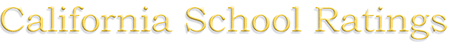# Alliance Margaret M. Bloomfield Technology Academy High 7907 Santa Fe Avenue Huntington Park, CA 90255

 CSR Rank: None Percentile: (2016) None1-d Compare to 2013 - Rank: None, API Score:

2016 CAASPP Test Score Details:
Standard
Met
Standard
Nearly Met
Standard
Standard
Not Met
- Asterisk "*", if present, indicates scores are not available (too few)
Dropouts:
90146
101 (0.75%)134
Student Ethnicity:
 Hispanic or Latino 99.48% African American 0.52%

Notes:
1. California School Ratings (CSR) computes percentiles in this way:
1. For a given grade level, all Math scores are put into an ordered list and a percentile is calculated for each score, based its position in the list.
2. For a given grade level, all English scores are put into an ordered list and a percentile is calculated for each score, based its position in the list.
3. Math and English percentiles from (a & b above) are weighted, based on the number of students who completed each type of test, to create a combined Math+English weighted percentile for each school.
4. The combined Math+English weighted percentiles are put into an ordered list for the particular type of school (elementary/middle/high school/K-12) and a percentile-within-the-school-type is calculated.
5. How percentiles work: the school percentile is a number between 0 and 100 that reflects the percentage of schools of the same type (elementary/middle/high school/K-12) in California that have an equal or lower combined Math+English weighted percentile (from 1-c above). For example, a school in the 70th percentile would have a combined weighted percentile that was equal to or better than 70% of the other schools of the same type.
2. The CSR Rank is determined by a school's percentile in comparison to other schools of the same type in California (from 1-d above). (1 is the worst, 10 is the best). Schools in the 90th percentile and above have rank 10, 80%-89.999% rank 9 and so on. A similar number of schools occupy each rank. * This rank is derived from data in the 2016 California Assessment of Student Performance and Progress (CAASPP).
3. Alternative Schools receive percentiles, but are not ranked